Test: Biasing In BJT Amplifier Circuits & Spread Spectrum

# Test: Biasing In BJT Amplifier Circuits & Spread Spectrum

Test Description

## 18 Questions MCQ Test Analog Circuits | Test: Biasing In BJT Amplifier Circuits & Spread Spectrum

Test: Biasing In BJT Amplifier Circuits & Spread Spectrum for Electronics and Communication Engineering (ECE) 2022 is part of Analog Circuits preparation. The Test: Biasing In BJT Amplifier Circuits & Spread Spectrum questions and answers have been prepared according to the Electronics and Communication Engineering (ECE) exam syllabus.The Test: Biasing In BJT Amplifier Circuits & Spread Spectrum MCQs are made for Electronics and Communication Engineering (ECE) 2022 Exam. Find important definitions, questions, notes, meanings, examples, exercises, MCQs and online tests for Test: Biasing In BJT Amplifier Circuits & Spread Spectrum below.
Solutions of Test: Biasing In BJT Amplifier Circuits & Spread Spectrum questions in English are available as part of our Analog Circuits for Electronics and Communication Engineering (ECE) & Test: Biasing In BJT Amplifier Circuits & Spread Spectrum solutions in Hindi for Analog Circuits course. Download more important topics, notes, lectures and mock test series for Electronics and Communication Engineering (ECE) Exam by signing up for free. Attempt Test: Biasing In BJT Amplifier Circuits & Spread Spectrum | 18 questions in 15 minutes | Mock test for Electronics and Communication Engineering (ECE) preparation | Free important questions MCQ to study Analog Circuits for Electronics and Communication Engineering (ECE) Exam | Download free PDF with solutions
 1 Crore+ students have signed up on EduRev. Have you?
Test: Biasing In BJT Amplifier Circuits & Spread Spectrum - Question 1

### (Q.1-Q.6) consider the figure shown below and answer the questions that proceed.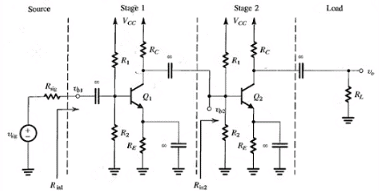For Vcc = 15V, R1 = 100 kΩ, R(E) = 3.9 kΩ, R(C) = 6.8 kΩ and ß = 100, determine the dc collector current for each transistor.

Detailed Solution for Test: Biasing In BJT Amplifier Circuits & Spread Spectrum - Question 1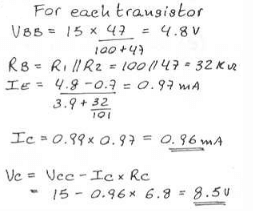Test: Biasing In BJT Amplifier Circuits & Spread Spectrum - Question 2

### For Vcc = 15V, R1 = 100 kΩ, R(E) = 3.9 kΩ, R(C) = 6.8 kΩ and ß = 100, determine the dc collector voltage for each transistor.

Detailed Solution for Test: Biasing In BJT Amplifier Circuits & Spread Spectrum - Question 2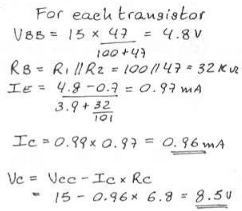Test: Biasing In BJT Amplifier Circuits & Spread Spectrum - Question 3

### Find R (in 1) for R (sig) = 5 kΩ.

Detailed Solution for Test: Biasing In BJT Amplifier Circuits & Spread Spectrum - Question 3

It is the parallel combination of the 32 kΩ resistor and 2.6 kΩ resistor respectively.

Test: Biasing In BJT Amplifier Circuits & Spread Spectrum - Question 4

Find Vb1/Vsig for R(sig) = 5 kΩ.

Detailed Solution for Test: Biasing In BJT Amplifier Circuits & Spread Spectrum - Question 4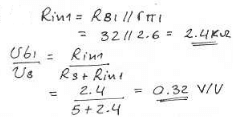Test: Biasing In BJT Amplifier Circuits & Spread Spectrum - Question 5

Find R (in 2).

Detailed Solution for Test: Biasing In BJT Amplifier Circuits & Spread Spectrum - Question 5

It is the parallel combination of the 32 kΩ resistor and 2.6 kΩ resistor respectively.

Test: Biasing In BJT Amplifier Circuits & Spread Spectrum - Question 6

Find Vb2/Vb1.

Detailed Solution for Test: Biasing In BJT Amplifier Circuits & Spread Spectrum - Question 6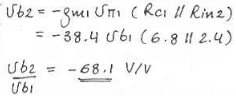Test: Biasing In BJT Amplifier Circuits & Spread Spectrum - Question 7

For Rl = 2 kΩ find Vo/Vb2.

Detailed Solution for Test: Biasing In BJT Amplifier Circuits & Spread Spectrum - Question 7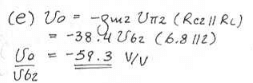Test: Biasing In BJT Amplifier Circuits & Spread Spectrum - Question 8

Find the overall voltage gain.

Detailed Solution for Test: Biasing In BJT Amplifier Circuits & Spread Spectrum - Question 8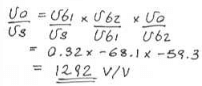Test: Biasing In BJT Amplifier Circuits & Spread Spectrum - Question 9

(Q.1-Q.3) A pseudo-noise (PN) sequence is generated using a feedback shift register of length m = 4. The chip rate is 107 chips per second.

Q. The PN sequence length is

Detailed Solution for Test: Biasing In BJT Amplifier Circuits & Spread Spectrum - Question 9

The PN sequence length is N = 2m – 1 = 16 – 1 = 15.

Test: Biasing In BJT Amplifier Circuits & Spread Spectrum - Question 10

The chip duration is

Detailed Solution for Test: Biasing In BJT Amplifier Circuits & Spread Spectrum - Question 10

Tc = 1/(107) or 0.1 µs.

Test: Biasing In BJT Amplifier Circuits & Spread Spectrum - Question 11

The period of PN sequence is

Detailed Solution for Test: Biasing In BJT Amplifier Circuits & Spread Spectrum - Question 11

The period of the PN sequence is T = NTc = 15 x 0.1 = 1.5 s

Test: Biasing In BJT Amplifier Circuits & Spread Spectrum - Question 12

A slow FH/MFSK system has the following parameters.
Number of bits per MFSK symbol = 4
Number of MFSK symbol per hop = 5
The processing gain of the system is

Detailed Solution for Test: Biasing In BJT Amplifier Circuits & Spread Spectrum - Question 12

PG = Wc/Rs = 5 x 4 = 20 or 26 db.

Test: Biasing In BJT Amplifier Circuits & Spread Spectrum - Question 13

A fast FH/MFSK system has the following parameters.
Number of bits per MFSK symbol = 4
Number of pops per MFSK symbol = 4
The processing gain of the system is

Detailed Solution for Test: Biasing In BJT Amplifier Circuits & Spread Spectrum - Question 13

PG = 4 x 4 = 16 or 12 db.

Test: Biasing In BJT Amplifier Circuits & Spread Spectrum - Question 14

(Q.6-Q.7) A rate 1/2 convolution code with dfrec = 10 is used to encode a data sequence occurring at a rate of 1 kbps. The modulation is binary PSK. The DS spread spectrum sequence has a chip rate of 10 MHz.

Q. The coding gain is

Detailed Solution for Test: Biasing In BJT Amplifier Circuits & Spread Spectrum - Question 14

0.5 x 10 = 5 or 7 db is the coding gain.

Test: Biasing In BJT Amplifier Circuits & Spread Spectrum - Question 15

The processing gain is

Detailed Solution for Test: Biasing In BJT Amplifier Circuits & Spread Spectrum - Question 15

PG = (107)/(2 x 1000) = 5000 or 37 db.

Test: Biasing In BJT Amplifier Circuits & Spread Spectrum - Question 16

(Q.8-Q.9) An FH binary orthogonal FSK system employs an m 15 stage liner feedback shift register that generates an ML sequence. Each state of the shift register selects one of L non over lapping frequency bands in the hopping pattern. The bit rate is 100 bits/s. The demodulator employ non coherent detection.[/expand]

Q.  If the hop rate is one per bit, the hopping bandwidth for this channel is

Detailed Solution for Test: Biasing In BJT Amplifier Circuits & Spread Spectrum - Question 16

The length of the shift-register sequence is L = 2m – 1215 = 32767 bits
For binary FSK modulation, the minimum frequency separation is 2/T, where 1/T is the symbol (bit) rate. The hop rate is 100 hops/sec. Since the shift register has L 32767 states and each state utilizes a bandwidth of 2/T = 200 Hz, then the total bandwidth for the FH signal is 6.5534 MHz.

Test: Biasing In BJT Amplifier Circuits & Spread Spectrum - Question 17

Suppose the hop rate is increased to 2 hops/bit and the receiver uses square law combining the signal over two hops. The hopping bandwidth for this channel is

Detailed Solution for Test: Biasing In BJT Amplifier Circuits & Spread Spectrum - Question 17

If the hopping rate is 2 hops/bit and the bit rate is 100 bits/sec, then, the hop rate is 200 hops/sec. The minimum frequency separation for orthogonality 2/T 400 Hz. Since there are N 32767 states of the shift register and for each state we select one of two frequencies separated by 400 Hz, the hopping bandwidth is 13.1068 MHz.

Test: Biasing In BJT Amplifier Circuits & Spread Spectrum - Question 18

In a fast FH spread spectrum system, the information is transmitted via FSK with non coherent detection. Suppose there are N = 3 hops/bit with hard decision decoding of the signal in each hop. The channel is AWGN with power spectral density 0.5No and an SNR 20 ~13 dB (total SNR over the three hops). The probability of error for this system is

Detailed Solution for Test: Biasing In BJT Amplifier Circuits & Spread Spectrum - Question 18

The total SNR for three hops is 20 ~ 13 dB. Therefore the SNR per hop is 20/3. The probability of a chip error with non-coherent detection is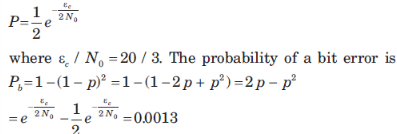## Analog Circuits

1 videos|17 docs|43 tests
 Use Code STAYHOME200 and get INR 200 additional OFF Use Coupon Code
Information about Test: Biasing In BJT Amplifier Circuits & Spread Spectrum Page
In this test you can find the Exam questions for Test: Biasing In BJT Amplifier Circuits & Spread Spectrum solved & explained in the simplest way possible. Besides giving Questions and answers for Test: Biasing In BJT Amplifier Circuits & Spread Spectrum, EduRev gives you an ample number of Online tests for practice

## Analog Circuits

1 videos|17 docs|43 tests